﻿ PIV Measurements to Study the Effect of the Reynolds Number on the Hydrodynamic Structure in a Baffled Vessel Stirred by a Rushton Turbine

### PIV Measurements to Study the Effect of the Reynolds Number on the Hydrodynamic Structure in a Baffl...

Zied Driss, Ahmed Kaffel, Bilel Ben Amira, Ghazi Bouzgarrou, Mohamed Salah AbidOPEN ACCESSPEER-REVIEWED

## PIV Measurements to Study the Effect of the Reynolds Number on the Hydrodynamic Structure in a Baffled Vessel Stirred by a Rushton Turbine

Zied Driss1,, Ahmed Kaffel1, Bilel Ben Amira1, Ghazi Bouzgarrou1, Mohamed Salah Abid1

1Laboratory of Electro-Mechanic Systems (LASEM), National School of Engineers of Sfax (ENIS), University of Sfax (US), Tunisia

### Abstract

Turbulent flow inside a cylindrical baffled stirred vessel is studied experimentally for different Reynolds numbers. A set of speed was selected ranging from 100 rpm to 350 rpm. These speeds gave high turbulence but without significant surface vortex formation. Vector field’s maps and contours of time averaged velocities, for both radial and axial components in the impeller stream of a vessel stirred by a Rushton turbine, were determined by means of 2D PIV technique. This study reveals the importance of choosing the whole flow field of the entire vessel in order to provide comprehensive understanding of the flow pattern and mixing conditions which is essential for reliable design.

### At a glance: Figures

123
Prev Next

• Driss, Zied, et al. "PIV Measurements to Study the Effect of the Reynolds Number on the Hydrodynamic Structure in a Baffled Vessel Stirred by a Rushton Turbine." American Journal of Energy Research 2.3 (2014): 67-73.
• Driss, Z. , Kaffel, A. , Amira, B. B. , Bouzgarrou, G. , & Abid, M. S. (2014). PIV Measurements to Study the Effect of the Reynolds Number on the Hydrodynamic Structure in a Baffled Vessel Stirred by a Rushton Turbine. American Journal of Energy Research, 2(3), 67-73.
• Driss, Zied, Ahmed Kaffel, Bilel Ben Amira, Ghazi Bouzgarrou, and Mohamed Salah Abid. "PIV Measurements to Study the Effect of the Reynolds Number on the Hydrodynamic Structure in a Baffled Vessel Stirred by a Rushton Turbine." American Journal of Energy Research 2, no. 3 (2014): 67-73.

 Import into BibTeX Import into EndNote Import into RefMan Import into RefWorks

### 1. Introduction

Stirring vessels are devices that find extensive applications particularly in chemical, food and biological process industries. Nowadays, numerical simulations are completed with experimental study to construct a better knowledge about the flow characteristics in a stirred vessel [1-8]. Various methods and techniques may be employed when achieving experimental study. Among these techniques, the non-intrusive are now widely encountered benefiting from the cutting-edge technology of optical visualization. To visualize flow, many researchers used Particle Image Velocimetry (PIV) as an innovative technique. PIV has emerged as a non-intrusive and efficient tool capable of measuring instantaneously the whole flow field overcoming the drawback of the laser doppler velocimetry (LDV) technique which permits the measurement of a single point only . Escudié and Liné  used PIV to determine the mean flow field, the turbulent fluctuations and turbulence characteristics produced by a Rushton turbine. Aubin et al.  studied the hydrodynamics of the liquid phase in an aerated vessel stirred with a six pitched blade turbine (PBT) using PIV measurements. Ranade et al.  used PIV results to validate computational fluid dynamics (CFD) simulations of gas-liquid flows in a tank stirred by a Rushton turbine. Khan et al.  employed a PIV technique to obtain angle-resolved fields of all three velocity components close to a 45° down pumping pitched-blade turbine operated at 300 rpm in an agitated vessel. Gabriele et al.  estimated local specific energy dissipation rates for up and down-pumping pitched blade turbines in a stirred tank using angle resolved PIV. A dual Rushton impeller stirred tank study was carried out by Chunmei et al.  using PIV measurements. Li et al.  used the PIV technique to calculate the velocity field generated by a Rushton turbine. Guida et al.  manipulated an experimental technique to calculate the flow distribution in a stirred tank containing coarse glass particles. These particles were suspended in water that is generated by a pitched blade turbine (PBT). Aubin et al.  used particle image velocimetry (PIV) technique to calculate the effect of the aerated agitator configuration in the mean velocity fields equipped by a pitched blade turbine. Guida et al.  used 2D PIV system to determine the azimuthal position effect of the measurement plane which is separated by 5 degree intervals from the adjacent one in a fully baffled vessel. Baldi and Yianneskis  used particle image velocimetry measurements to calculate the mean velocities, Reynolds stresses and the turbulence energy dissipation rate in a stirred vessel by a Rushton turbine.

Most of previous studies focused on a measurement area close to the tip of the blade [10-18]. The present work focuses on the effect of Reynolds number on the time averaged velocity.

### 2. Geometrical Arrangement

A schematic presentation of the flat-bottom vessel equipped by a Rushton turbine is given in Figure 1. The measurements were performed in a standard configuration stirred vessel of diameter D=0.3 m and equipped with four equally-spaced baffles made of glass of width equal to b=D/10. The vessel made from Plexiglas (5 mm thick) was filled with tap water and placed inside a Plexiglas squared tank filled with water in order to minimize optical distortion over the cylindrical surface of the vessel. The aerated vessel was stirred by a standard Rushton turbine with a diameter d=D/3. The bottom clearance y was equal to the impeller diameter (y=d). The blade length was equal to l=d/4, and the blade height was equal to h=d/5.

### 3. Mathematical Formulation

To analyze different types of fluctuations induced by the Rushton turbine, an angle resolved measurement has been conducted. An encoder mounted on the shaft of the impeller enables the velocity field measurement to be synchronized. Thus, the three components of the velocity vector can be evaluated. The instantaneous velocity field is decomposed into average velocity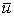and fluctuant velocity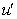: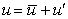(1)

This is commonly known as Reynolds decomposition. Where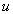is the instantaneous velocity,is the ensemble average velocity calculated for each plane as follow: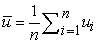(2)

Where n is the number of acquisitions, and the over-bar denotes the time average.

The componentis the fluctuant velocity directly obtained by subtracting the average velocity from the instantaneous velocity: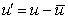(3)

It is wide clear that the fluctuant velocity changes in sign with time evolution either positive or negative. To characterize turbulence strength independently whatever the sign, the term rms velocity calculated for the radial velocity are as follow: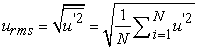(4)

The subscript rms stands for "root-mean-square". In mathematics, rms velocity is also known as quadratic mean. A larger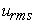indicates a higher level turbulence.

The turbulent kinetic energy field can be calculated using the formula: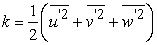(5)

With 2-D PIV data, there is no knowledge of the third rms velocity component (usually tangential) and k can only be estimated using a pseudo-isotropic assumption defined as follows: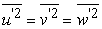(6)

This yields the following simplified formula: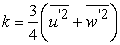(7)

This assumption was verified experimentally by Khan et al.  for a PBT using stereoscopic PIV data. In the later discussion. The turbulent kinetic energy is normalized using the square of the impeller tip speed.

The dissipation rate of the turbulent kinetic energy could be estimated from dimensional relations: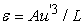Where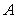is a constant,the turbulence level in the main flow direction and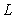a characteristic length scale.

The dissipation rate of the turbulent kinetic energy could be obtained directly from PIV output data. PIV enables the measurement of instantaneous velocities over an area, and therefore the instantaneous spatial gradients can be calculated and direct estimation of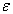can be performed. In cylindrical coordinates it is calculated as follows: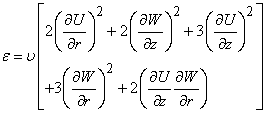(8)

Where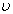is the effective viscosity, r and z are the coordinate system. This time-averaged equation contains unknown terms. In our case, 2-D PIV measurements have been conducted. The local isotropy hypothesis has been employed to overcome the lack of information of the remaining terms of the ε equation, where only twelve terms could be determined.

The use of the isotropic approximation to calculate velocity gradients evokes two concepts that must be clarified, the homogeneous turbulence and the isotropic turbulence. The distinction between these two concepts is very important. In fact, “Homogeneous turbulence” is defined as statistically invariant to shifts of the coordinate system, and “isotropic turbulence” is additionally invariant to rotations and reflections.

The Reynolds number Re is defined as follow: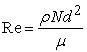(9)

Where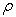is the fluid density, N is the impeller speed, d is the diameter of the impeller and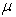is the fluid viscosity.

### 4. Measuring Technique

The flow was seeded with neutrally buoyant 20 µm polyamide particles (Figure 2). The acquisition system for the PIV measurements consists of a double-cavity 30 mJ per pulse at wavelength 532 nm Nd:YAG laser form QUANTEL and a PCO camera of 1600 x1200 pixels. The laser beam is converted to a sheet of laser of thickness 1.5 mm to provide a near two-dimensional analysis of the flow. The PIV image pairs are cross-correlated with a 32×32 interrogation window and 50 % overlap. The time between pulses was chosen to ensure that the maximum displacement does not exceed a quarter of the side of the interrogation area as described by Keane and Adrian  and Adrian . Thus, the exposure time delay between images was set to 0.2 ms. This yielded a spatial resolution of 0.25 mm/pixels. A gray scale recorded image which represents the scattered light of embedded tracer particles in water flow mixed by a Rushton turbine derived from the experimental work at the laboratory. All distances are normalized with the impeller diameter d and the velocities are normalized with the blade tip velocity Utip. The reference coordinate system is fixed at the bottom of the impeller axis and the impeller rotation is clockwise.

### 5. Results and Discussion

Impeller speeds of N=100 rpm, N=150 rpm, N=200 rpm, N=250 rpm, N=300 rpm and N=350 rpm were used corresponding to Reynolds numbers of Re=17000, Re=25000, Re=33000, Re=42000, Re=50000 and Re=58000. In these conditions, the blade tip-velocities values vary between Utip=0.52 m.s-1 and Utip=1.83 m.s-1. The measurement area covers the whole flow field along a plane over the right half of the vessel.

5.1. Velocity Field

Figure 3 presents the time-averaged velocity field between two blades over 170 image pairs of instantaneous velocity fields for impeller speed varying over Reynolds numbers ranging from Re=17000 to Re=58000. According to these results, it is clear that the Reynolds number has a direct effect on the intensify values. However, the same distribution of the velocity field has been observed. In fact, the Rushton turbine generates two main vertical motions, above and below the impeller region. The jet flow coming off the impeller blades streams towards the wall and divides into two flows under the influence of the vessel wall. The first one is downward along the wall to the bottom of the vessel, then back to the impeller region. The second rises along the wall to the top of the vessel and back to the impeller region, forming double loop. The vector plot shows that the predominant direction of the stream is in the radial direction. For low Reynolds numbers, the flow motion indicates a slowdown in the upper section of the vessel. The flow tends to be strong and fully developed at high Reynolds numbers as shown in Figure 4.e and Figure 4.f. The highest values are close to the tip of the blade and decreasing with radial distance from the blade. Regions of slower flow in the upper section of the planar measurement window begin to develop as the field progresses with increased impeller speed.

The contour plot values normalized by Utip as depicted in Figure 4, show maximum magnitudes located at the tip of the blade for different Reynolds numbers of Re=17000, Re=25000, Re=33000, Re=42000, Re=50000 and Re=58000. These values increase with rotational impeller speed of the stream jet in the radial direction along the impeller jet-stream. Indeed, the same distribution of the average radial velocity has been observed.

5.3. Average Axial Velocity

Figure 5 shows the contour plots of the average axial velocity for different Reynolds numbers of Re=17000, Re=25000, Re=33000, Re=42000, Re=50000 and Re=58000. According to these results, it is clear that the Reynolds number has a direct effect on the intensify values. However, the same distribution of the average axial velocity has been observed. The above mentioned outward motion is divided into two flows under the influence of the vessel wall. One is downward (blue color) along the wall, to the bottom of the vessel, then back to the impeller region. The other rises along the wall (red color), to the top of the vessel and back to the impeller region. A comparison between radial and axial contour plots reveals the well known radial character of this type of turbines. The intensity of the axial component in the upward direction near the vessel walls may be up to 50 % of the blade tip velocity in the impeller stream region. The absence of velocity vectors at the top of the upper section of the vessel is caused by the aerated surface of the flow which leads to the loss of correlation between particle image pairs.

5.4. Axial Profiles of the Average Radial Velocity

In order to study the evolution of the radial discharge stream in the impeller region, a superposition of axial profiles of radial averaged velocities has been made. As expected, it is observable in Figure 6 that the maximum value is located at the dimensionless axial position equal to z=0.66 which corresponds to the bottom clearance normalized by the tank diameter. For the Reynolds number Re=17000, the maximum radial dimensionless velocity is equal to U=0.213 and reaches U=0.54 for Re=58000. It should be noted that the influence of the baffle facing the measurement area is unavoidable with the given configuration since it reduces significantly the velocity especially at its borders where the imaging of suspended particles is extremely difficult and consequently the correlation is affected.

Figure 7. Evolution of the maximum value of the turbulent kinetic energy
5.5. Axial Profile of the Turbulent Kinetic Energy

Figure 7 shows the evolution of the turbulent kinetic energy occurring at the impeller tip at measurement planes just behind the leading blade. According to these results, it is clear that the maximum values of the turbulent kinetic energy lie in the range of angles between 15° and 30° behind the leading blade. The maximum value increases as the angle from the blade increases, where the slope of the curve rapidly increases from k=0.54 at 10° to reach k=1.3 at 15°. Then, it drops to reach k=0.57 at 55°. Meanwhile, the locus of the maximum levels slightly moves away from impeller region to reach a radial position equal to r=0.45. In fact, the locus of the highest values seems to follow the trailing vortices. During this movement, the locus of maximum values is being stretched along the radial direction. These results are in good agreement with several publications reporting the presence of this aspect [10, 11, 12]. Such study may be completed by taking measurements in the horizontal plane in order to follow the direction and the expanse of the trailing vortices relative to each angle.

### 6. Conclusion

In this paper, we are interested on the experimental study of the hydrodynamic structure of a cylindrical baffled stirred vessel equipped with a Rushton turbine. Measurements were performed at different Reynolds numbers via 2D PIV technique. The behavior of the flow in a cylindrical mixing vessel has been studied in terms of averaged velocities. Particularly, vector field’s maps and contours of time averaged velocities, for both radial and axial components are presented in the impeller stream of a vessel stirred. In these conditions, it has been noted that the Reynolds number has a direct effect on the intensify values. However, the same distribution of the whole flow circulation pattern has been observed. The choice of the whole vessel as measurement domain to be recorded has been efficient in determining the whole flow circulation pattern. It reveals some specific measurements and particularities to be considered even though it reduces the spatial resolution needed to achieve further studies of turbulence. Indeed, this work should be followed by 3D PIV analysis using stereoscopic arrangement capable of capturing the third component of velocity.

### Nomenclature

A: constant (dimensionless)

b: width of the baffles (m)

D: diameter of the cylindrical tank (m)

d: diameter of the impeller (m)

H: water depth (m)

k: turbulent kinetic energy (dimensionless)

L: characteristic length scale (dimensionless)

N: Impeller speed (rpm)

n : acquisitions number

Re: Reynolds number

rms: root mean square

t: times (s)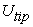: tip-velocity (m.s-1): instantaneous velocity (m.s-1): rms velocity (m.s-1): average velocity (m.s-1): fluctuant radial velocity (m.s-1)

V: tangential velocity component (dimensionless)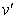: fluctuant tangential velocity (m.s-1)

W: axial velocity component (dimensionless)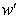: fluctuant axial velocity (m.s-1)

y: clearance of the turbine in the tank (m)

z: axial coordinate (dimensionless)

ε: dissipation rate of the turbulent kinetic energy (dimensionless)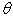: angular position (°): fluid viscosity (Pa.s): effective viscosity (dimensionless): fluid density (kg.m-3)

### References

  Driss, Z., Bouzgarrou, G., Chtourou, W., Kchaou, H., Abid, M. S., “Computational studies of the pitched blade turbines design effect on the stirred tank flow characteristics,” European Journal of Mechanics B/Fluids, 29. 236-245. 2010.In article CrossRef  Driss, Z., Karray, S., Chtourou, W., Kchaou, H., Abid, M.S., “A Study of Mixing Structure in Stirred Tanks Equipped with Multiple Four-Blade Rushton Impellers,” Archive of Mechanical Engineering, 59. 53-72. 2012.In article  Karray, S., Driss, Z., Kchaou, H., Abid, M.S., “Numerical simulation of fluid-structure interaction in a stirred vessel equipped with an anchor impeller,” Journal of Mechanical Science and Technology (JMST), 25. 1749-1760. 2011.In article CrossRef  Ammar, M., Chtourou, W., Driss, Z., Abid, M.S., “Numerical investigation of turbulent flow generated in baffled stirred vessels equipped with three different turbines in one and two-stage system,” Energy, 36 (8). 5081-5093. 2011In article CrossRef  Ammar, M., Chtourou, W., Driss, Z., Abid, M.S., “Modelling of the turbulent flow generated with a PBT turbine in baffled stirred vessels using steady and unsteady models,” Science Academy Transactions on Renewable Energy Systems Engineering and Technology, 1 (4). 134-143. 2011.In article  Driss, Z., Karray, S., Kchaou, H., Abid, M.S., “Computer simulations of laminar flow generated by an anchor blade and a Maxblend impellers,” Science Academy Transactions on Renewable Energy Systems Engineering and Technology, 1 (3). 68-76. 2011.In article  Driss, Z., Karray, S., Kchaou, H., Abid, M.S., “CFD simulation of the laminar flow in stirred tanks generated by double helical ribbons and double helical screw ribbons impellers,” Cent. Eur. J. Eng., 1 (4), 413-422. 2011.In article CrossRef  Driss, Z., Bouzgarrou, G., Kaffel, A., Abid, M.S., “Experimental Study of the Seeding Concentration on the PIV Measurements Applied to a Stirred Vessel Equipped by a Rushton Turbine,” International Journal of Mechanics and Applications, 2 (5), 93-97. 2012.In article CrossRef  Suzukawa, K., Mochizuki, S., Osaka, H., “Effect of the attack angle on the roll and trailing vortex structures in an agitated vessel with a paddle impeller,” Chemical Engineering Science, 61. 2791-2798. 2006In article CrossRef  Escudié, R., Liné, A., “Experimental analysis of hydrodynamics in a radially agitated tank,” AIChE Journal, 49 (3). 585-603. 2003.In article CrossRef  Aubin, J., Sauze, N.L., Bertrand, J., Fletcher D.F., Xuereb, C., “PIV measurements of flow in an aerated tank stirred by a down and an up-pumping axial flow impellers,” Chemical Engineering Science, 28. 447-456. 2004.In article  Ranade, V.V., Perrard, M., Xuereb, C., Sauze, N.L., Bertrand, J., “Influence of gas flow rate on the structure of trailing vortices of a rushton turbine: PIV measurements and CFD simulations,” Institution of Chemical Engineers Trans IChemE, 79. 957-964. 2001.In article CrossRef  Khan, F.R., Rielly, C.D., Brown, D.A.R., “Angle-resolved stereo-PIV measurements close to a down-pumping pitched-blade turbine,” Chemical Engineering Science 61. 2799-2806. 2006.In article CrossRef  Gabriele, A., Nienow, A.W., Simmons, M.J.H., “On the quantification of energy dissipation in the impeller stream of a stirred vessel from fluctuating velocity gradient measurements,” Chemical Engineering Science, 64. 126-143. 2009.In article CrossRef  Chunmei, P., Jian, M., Xinhong L., Zhengming, G., “Investigation of fluid flow in a dual Rushton impeller stirred tank using particle image velocimetry,” Chinese Journal of Chemical Engineering, 16 (5). 693-699. 2008.In article CrossRef  Li, Z., Bao, Y., Gao, Z., “PIV experiments and large eddy simulations of single-loop flow fields in Rushton turbine stirred tanks,” Chemical Engineering Science, 66. 1219-1231. 2011.In article CrossRef  Guida, A., Nienow, A.W., Barigou, M., “PEPT measurements of solid-liquid flow field and spatial phase distribution in concentrated mono disperse stirred suspensions,” Chemical Engineering Science, 65. 1905-1914. 2010.In article CrossRef  Aubin, J., Sauze, N.L., Bertran, J., Fletcher, D.F., Xuereb, C., “PIV measurements of flow in an aerated tank stirred by a down and an up-pumping axial flow impeller,” Experimental Thermal and Fluid Science, 28. 447-456. 2004.In article CrossRef  Guida, A., Nienow, A.W., Barigou, M., “The effects of the azimuthal position of the measurement plane on the flow parameters determined by PIV within a stirred vessel,” Chemical Engineering Science, 65. 2454-2463. 2010.In article CrossRef  Baldi, S., Yianneskis, M., “On the quantification of energy dissipation in the impeller stream of a stirred vessel from fluctuating velocity gradient measurements,” Chemical Engineering Science, 59. 2659-2671. 2004.In article CrossRef  Keane, R.D., Adrian, R.J., Theory of cross-correlation analysis of PIV images, Appl. Sci. Res., 49. 191-215. 1992.In article CrossRef  Adrian, R.J., Twenty years of particle image velocimetry, “Experiments in Fluids,” 39. 159-169. 2005.In article CrossRef